# Calculus 2 : Concepts of Convergence and Divergence

## Example Questions

← Previous 1

### Example Question #1 : Concepts Of Convergence And Divergence

One of the following infinite series CONVERGES. Which is it?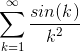None of the others converge.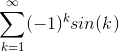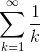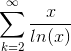Explanation:converges due to the comparison test.

We start with the equation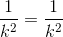. Since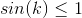for all values of k, we can multiply both side of the equation by the inequality and get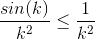for all values of k. Since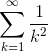is a convergent p-series with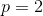hence also converges by the comparison test.

### Example Question #18 : Introduction To Series In Calculus

Determine the nature of the following series having the general term: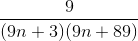The series is convergent.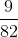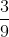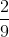The series is convergent.

Explanation:

We will use the Limit Comparison Test to show this result.

We first denote the genera term of the series by: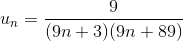and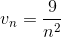.

We have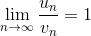and the series have the same nature .

We know that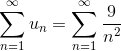is convergent by comparing the integral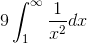which we know is convergent.

Therefore by the Limit Comparison Test.

we have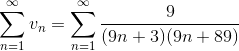.

### Example Question #19 : Introduction To Series In Calculus

If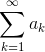converges, which of the following statements must be true?

The limit of the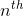partial sums as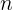approaches infinity is zero.

For some large value of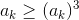.

None of the other answers must be true.

The limit of theterm asapproaches infinity is not zero.

For some large value of.

Explanation:

If the series converges, then we know the terms must approach zero. At some point, the terms will be less than 1, meaning when you take the third power of the term, it will be less than the original term.

Other answers are not true for a convergent series by theterm test for divergence.

In addition, the limit of thepartial sums refers to the value the series converges to. A convergent series need not converge to zero. The alternating harmonic series is a good counter example to this.

### Example Question #58 : Ap Calculus Bc

Which of following intervals of convergence cannot exist?For any, the interval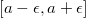for some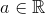For any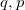such that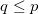, the interval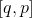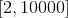Explanation: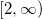cannot be an interval of convergence because a theorem states that a radius has to be either nonzero and finite, or infinite (which would imply that it has interval of convergence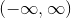). Thus,can never be an interval of convergence.

### Example Question #21 : Introduction To Series In Calculus

Which of the following statements is true regarding the following infinite series?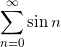The series converges because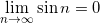The series diverges, by the divergence test, because the limit of the sequence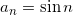does not approach a value as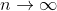The series diverges to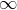.

The series diverges because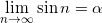for some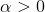and finite.

The series diverges, by the divergence test, because the limit of the sequencedoes not approach a value asExplanation:

The divergence tests states for a series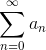, if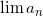is either nonzero or does not exist, then the series diverges.

The limit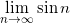does not exist, so therefore the series diverges.

### Example Question #22 : Introduction To Series In Calculus

Determine whether the following series converges or diverges: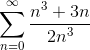The series converges.

The series diverges.

The series conditionally converges.

The series converges.

Explanation:

To prove the series converges, the following must be true:

If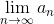converges, thenconverges.

Now, we simply evaluate the limit: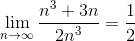The shortcut that was used to evaluate the limit as n approaches infinity was that the coefficients of the highest powered term in numerator and denominator were divided.

The limit approaches a number (converges), so the series converges.

### Example Question #64 : Ap Calculus Bc

Determine whether the following series converges or diverges. If it converges, what does it converge to?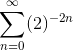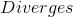Explanation:

First, we reduce the series into a simpler form.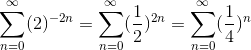We know this series converges because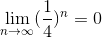By the Geometric Series Theorem, the sum of this series is given by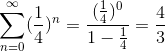### Example Question #23 : Introduction To Series In Calculus

Determine whether the following series converges or diverges. If it converges, what does it converge to?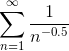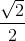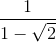Explanation:

Notice how this series can be rewritten as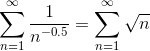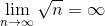Therefore this series diverges.

### Example Question #1 : Sequences & Series

There are 2 series,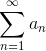and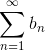, and they are both convergent. Is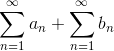convergent, divergent, or inconclusive?

Divergent

Convergent

Inconclusive

Convergent

Explanation:

Infinite series can be added and subtracted with each other.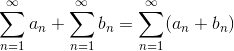Since the 2 series are convergent, the sum of the convergent infinite series is also convergent.

Note: The starting value, in this case n=1, must be the same before adding infinite series together.

### Example Question #2 : Sequences & Series

You have a divergent series, and you multiply it by a constant 10. Is the new series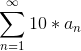convergent or divergent?

Inconclusive

Convergent

Divergent

Divergent

Explanation:

This is a fundamental property of series.

For any constant c, ifis convergent then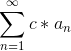is convergent, and ifis divergent,is divergent.is divergent in the question, and the constant c is 10 in this case, sois also divergent.

← Previous 1